1597724040

# Convolution layers: summary and overview

Convolution layers are fundamental building blocks of computer vision architectures. Neural networks employing convolutions layers are employed in wide ranging applications in Segmentation, Reconstruction, Scene Understanding, Synthesis, Object detection.

The goal of this post is to provide a summary and overview of advanced convolution layers and techniques which have emerged in the recent literature. We start with basics of convolution, for completeness, however, more rigorous explanations can be obtained from references within.

Convolution: Mathematically speak convolution is an “operation” performed to combine two signals into one, below is an illustration from wikipedia which highlights convolution between two functions/signals f(t) and f(t-z). Convolution to obtain the (f*g)(t)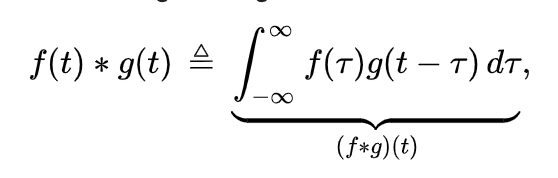Convolution from wikipedia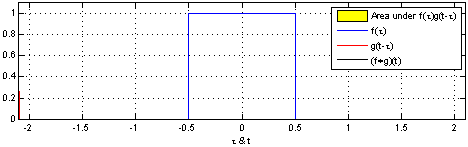The main convolution operations in deep learning are

1. “2D” Convolution:

Pictorially we convolve “slide” a kernel (green size) over an image (blue) and learn weights for these kernels. This kernel’s spatial extent (F) is 3 and filters i.e. depth of the kernel is 1, therefore number of weights are 331=9. We can skip pixels by “stride” and pad regions our original image, here the stride is 0

#neural-networks #artificial-intelligence #machine-learning #computer-vision #deep learning

## Buddha Community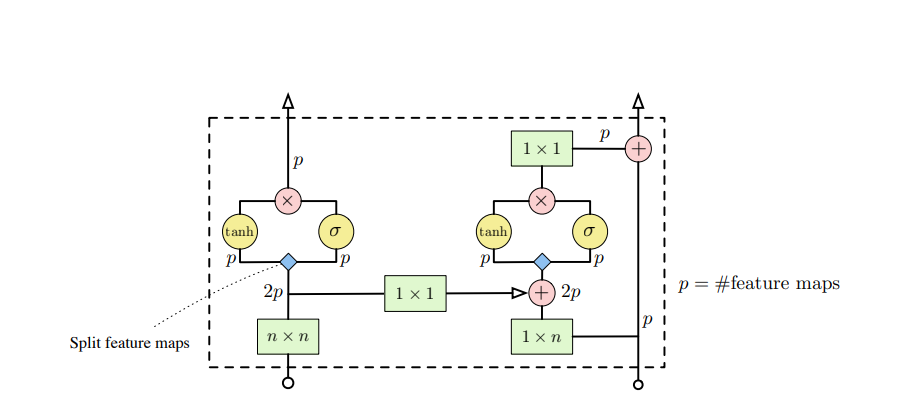1597724040

## Convolution layers: summary and overview

Convolution layers are fundamental building blocks of computer vision architectures. Neural networks employing convolutions layers are employed in wide ranging applications in Segmentation, Reconstruction, Scene Understanding, Synthesis, Object detection.

The goal of this post is to provide a summary and overview of advanced convolution layers and techniques which have emerged in the recent literature. We start with basics of convolution, for completeness, however, more rigorous explanations can be obtained from references within.

Convolution: Mathematically speak convolution is an “operation” performed to combine two signals into one, below is an illustration from wikipedia which highlights convolution between two functions/signals f(t) and f(t-z). Convolution to obtain the (f*g)(t)Convolution from wikipediaThe main convolution operations in deep learning are

1. “2D” Convolution:

Pictorially we convolve “slide” a kernel (green size) over an image (blue) and learn weights for these kernels. This kernel’s spatial extent (F) is 3 and filters i.e. depth of the kernel is 1, therefore number of weights are 331=9. We can skip pixels by “stride” and pad regions our original image, here the stride is 0

#neural-networks #artificial-intelligence #machine-learning #computer-vision #deep learning1596633180

## Deriving convolution from first principles

TL;DR:_ Have you even wondered what is so special about convolution? In this post, I derive the convolution from first principles and show that it naturally emerges from translational symmetry._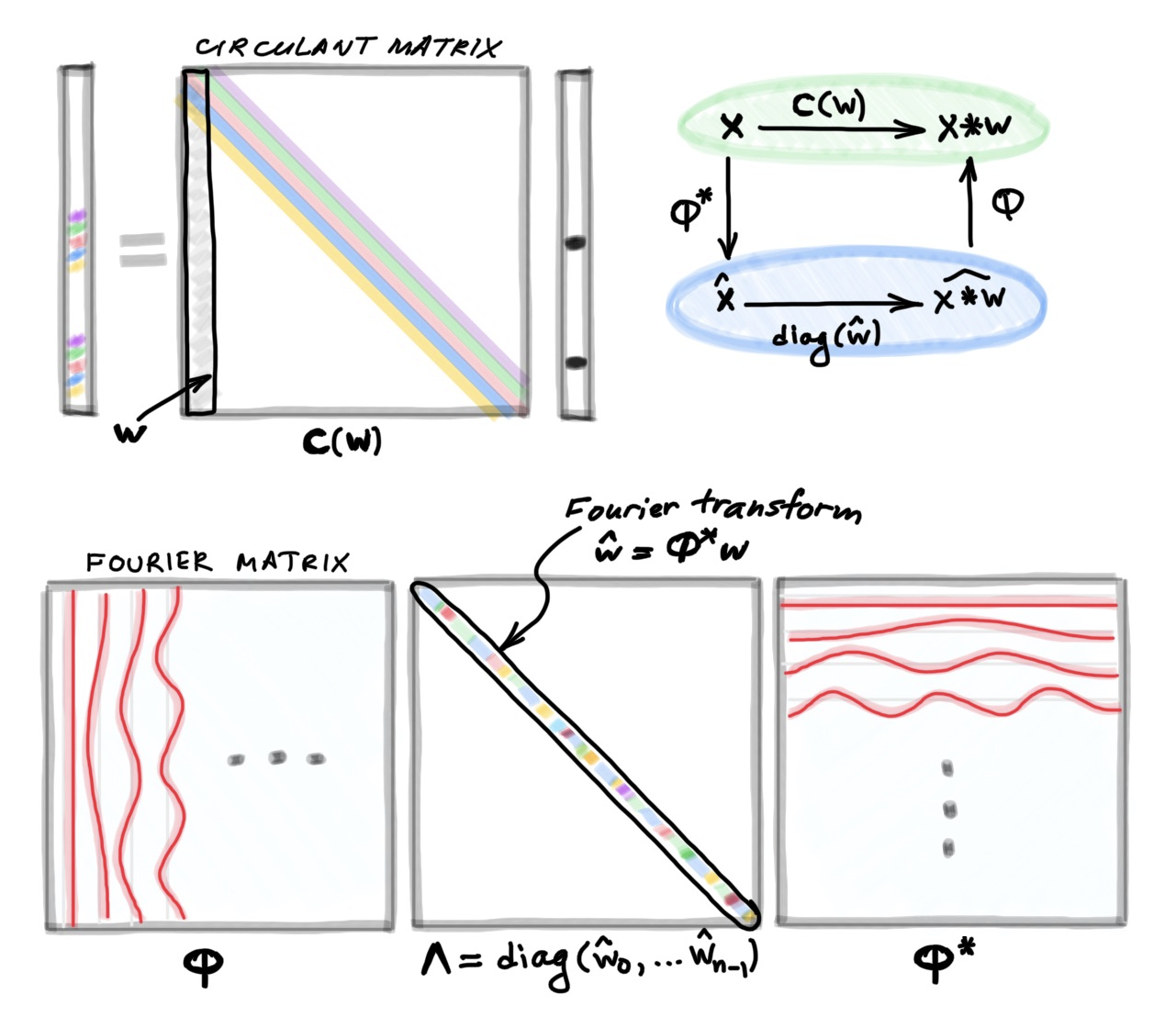La connoissance de certains principes supplée facilement à la connoissance de certains faits. (Claude Adrien Helvétius)

D

uring my undergraduate studies, which I did in Electrical Engineering at the Technion in Israel, I was always appalled that such an important concept as convolution  just landed out of nowhere. This seemingly arbitrary definition disturbed the otherwise beautiful picture of the signal processing world like a grain of sand in one’s eye. How nice would it be to have the convolution emerge from first principles rather than have it postulated! As I will show in this post, such first principles are the notion of translational invariance or symmetry.

Let me start with the formula taught in basic signal processing courses defining the discrete convolution  of two n-dimensional vectors x and w:Here, for convenience, I assume that all the indices run from zero to _n_−1 and are modulo n; it is convenient to think of vectors as defined on a circle. Writing the above formula as a matrix-vector multiplication leads to a very special matrix that is called circulant: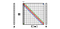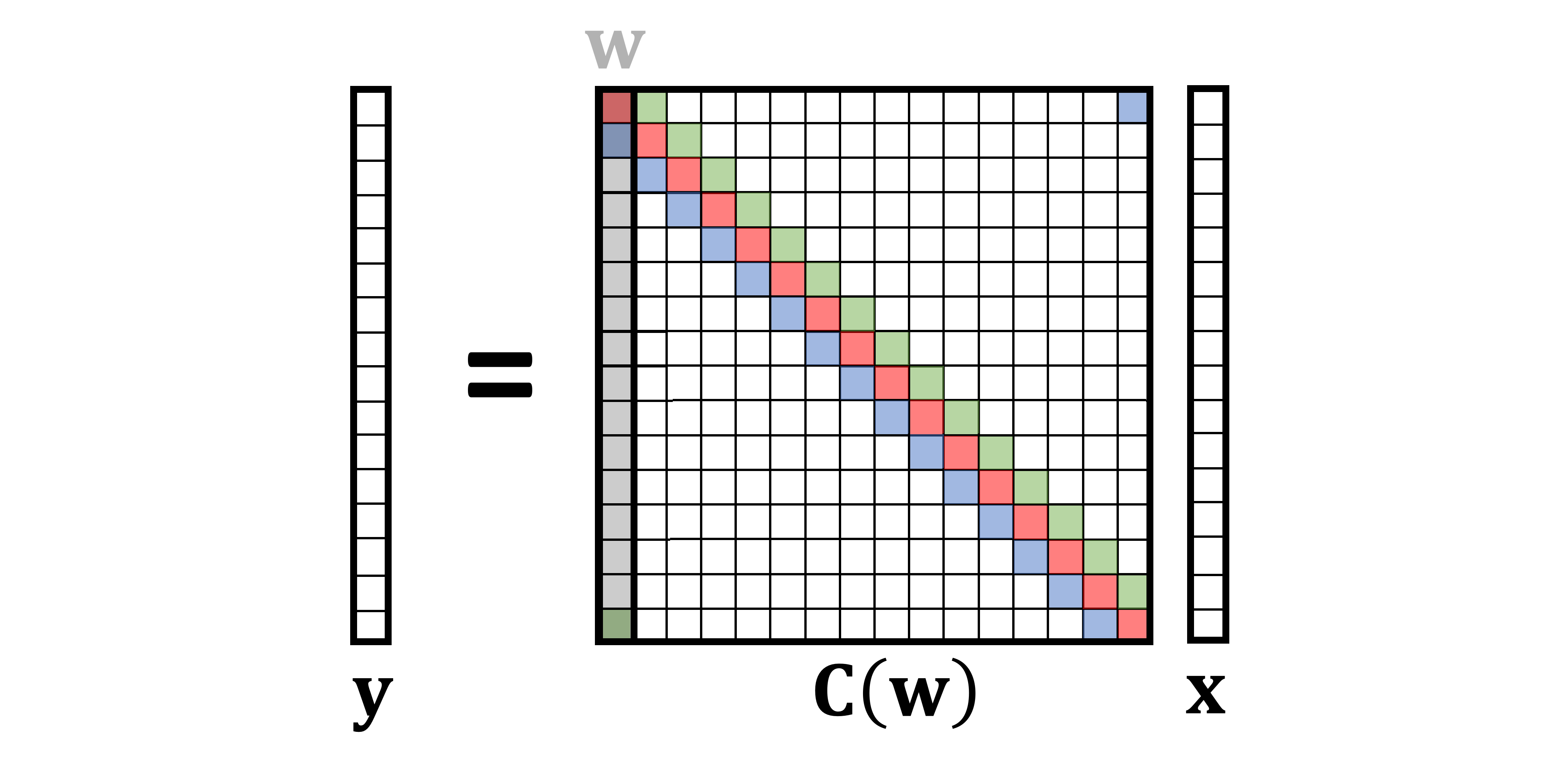A circulant matrix has multi-diagonal structure, with elements on each diagonal having the same value. It can be formed by stacking together shifted (modulo n) versions of a vector ; for this reason, I use the notation C(w) referring to a circulant matrix formed by the vector w. Since any convolution xwcan beequivalently represented as a multiplication by the circulant matrix C(w)x, I will use the two terms interchangeably.

One of the first things we are taught in linear algebra is that matrix multiplication is non-commutative, i.e.,in general, ABBA. However, circulant matrices are very special exception:

Circulant matrices commute,

or in other words, C(w)C(u)=C(u)C(w). This is true for any circulant matrix, or any choice of u and w. Equivalently, we can say that the convolution is a commutative operation, xw=wx.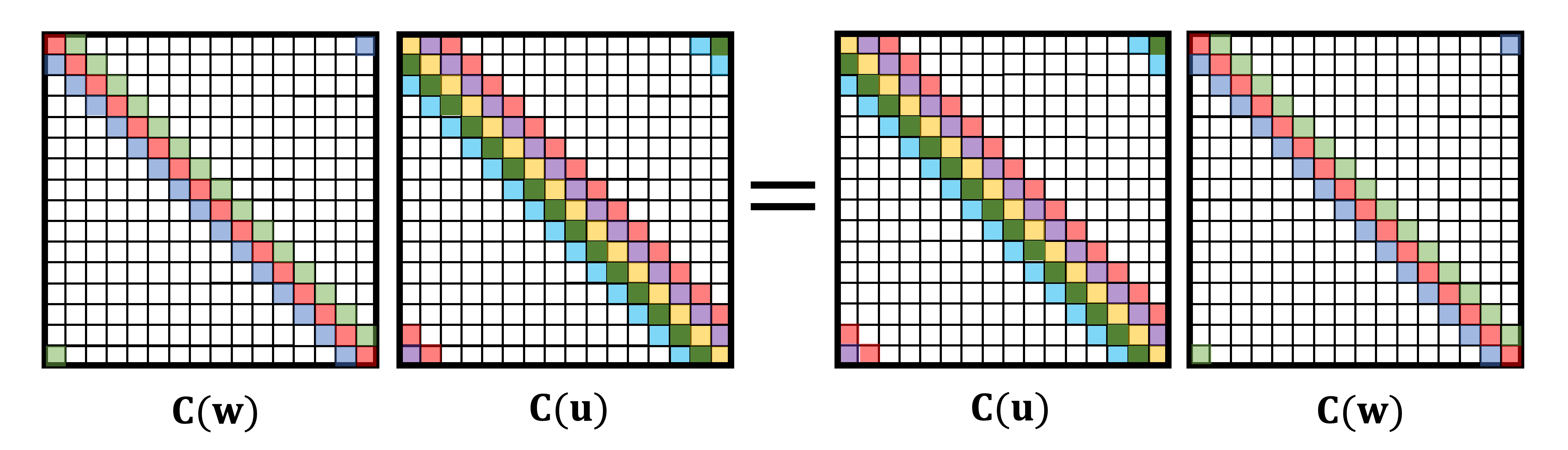A particular choice of w=[0,1,0…,0] yields a special circulant matrix that shifts vectors to the right by one position. This matrix is called the (right) _shift operator _ and denoted by S. The transpose of the right shift operator is the left shift operator. Obviously, shifting left and then right (or vice versa) does not do anything, which means S is an orthogonal matrix: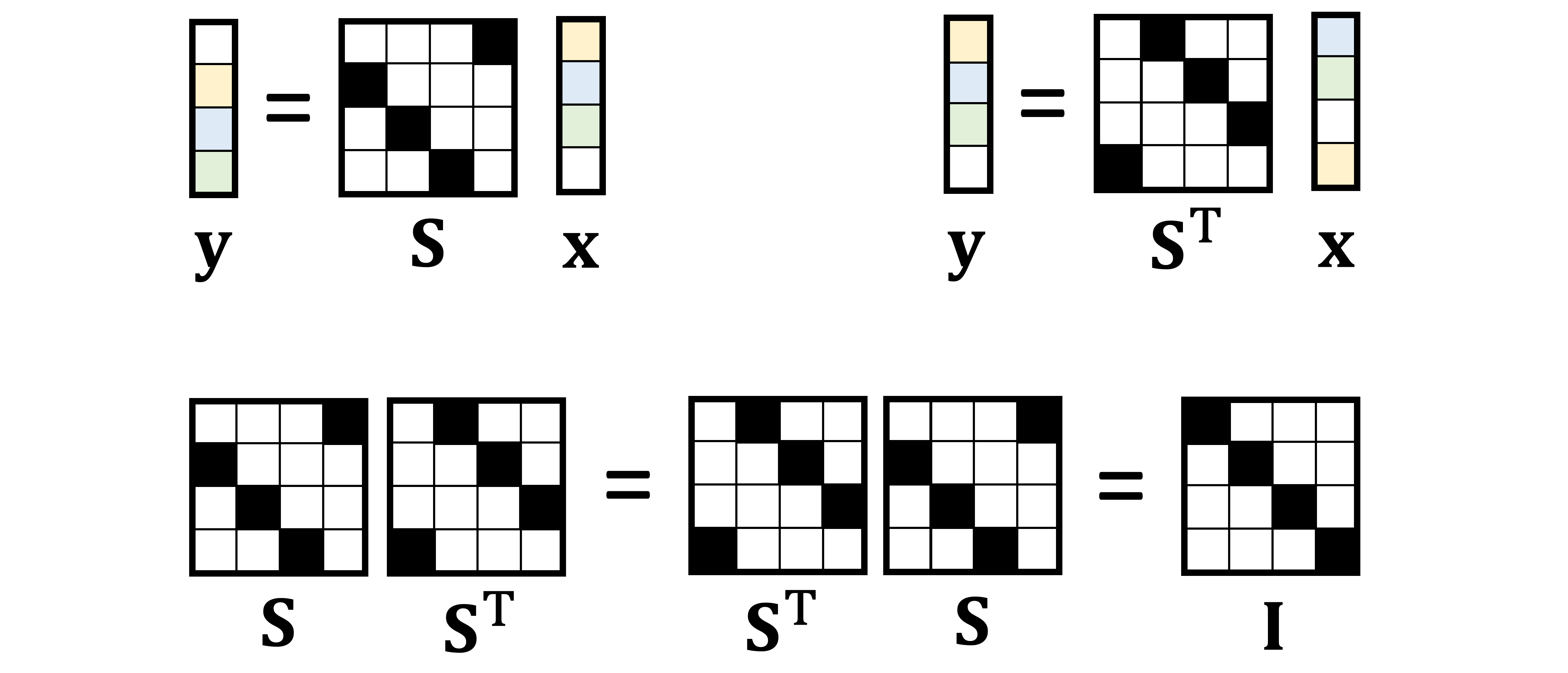Circulant matrices can be characterised by their commutativity property. It appears to be sufficient to show only commutativity with shift (Lemma 3.1 in ):

A matrix is circulant if and only if it commutes with shift.

The first direction of this “if and only if” statement leads to a very important property called translation or _shift equivariance _: the convolution’s commutativity with shift implies that it does not matter whether we first shift a vector and then convolve it, or first convolve and then shift — the result will be the same.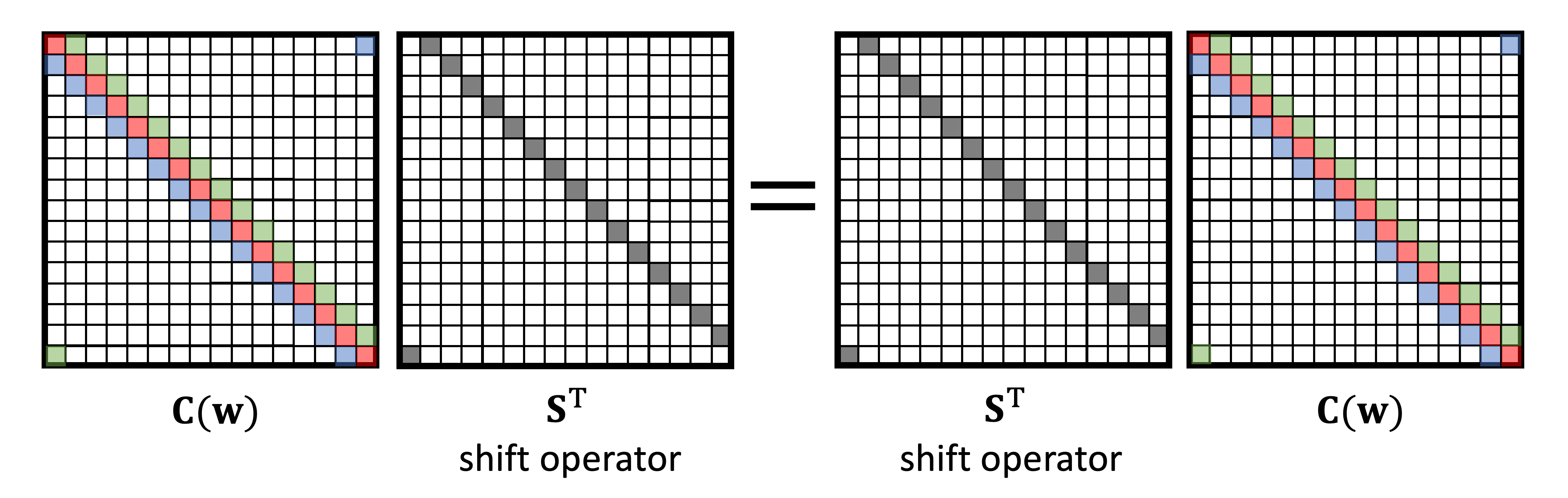The second direction allows us to define convolution as the shift-equivariant linear operation: in order to commute with shift, a matrix must have the circulant structure. This is exactly what we aspired to from the beginning, to have the convolution emerge from the first principles of translational symmetry . Instead of being given a formula of the convolution and proving its shift equivariance property, as it is typically done in signal processing books, we can start from the requirement of shift equivariance and arrive at the formula of the convolution as the only possible linear operation satisfying it.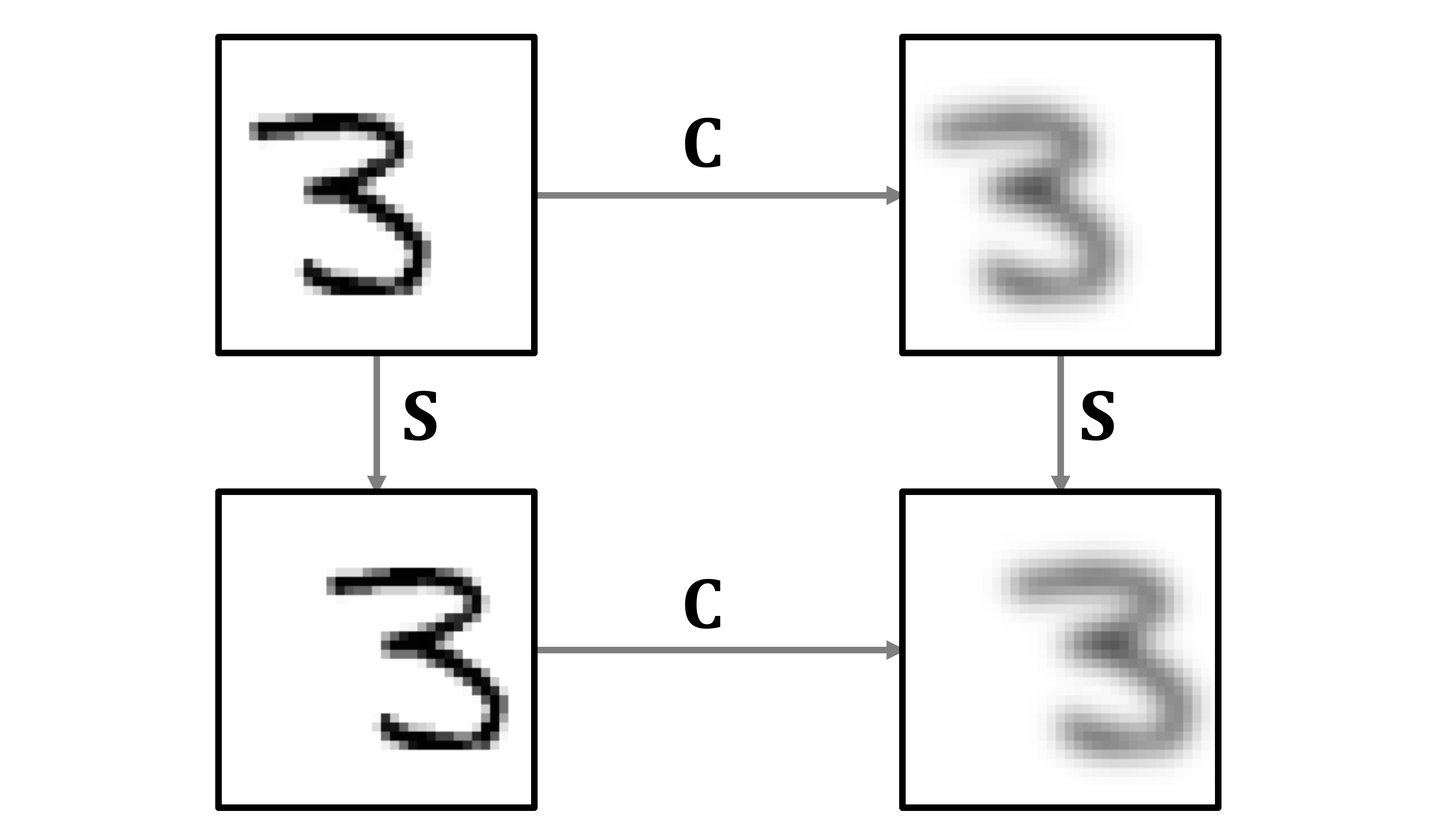Illustration of shift equivariance as the interchangeability of shift and blur operations.

A nother important fact taught in signal processing courses is the connection between the convolution and the Fourier transform . Here as well, the Fourier transform lands out of the blue, and then one is shown that it diagonalises the convolution operation, allowing to perform convolution of two vectors in the frequency domain as element-wise product of their Fourier transforms. Nobody ever explains where these sines and cosines come from and what is so special about them.

#deep-learning #convolutional-neural-net #data-science #machine-learning #convolution #deep learning1597277640

## Deriving convolution from first principles

##### Basics Of Deep Learning

TL;DR:_ Have you even wondered what is so special about convolution? In this post, I derive the convolution from first principles and show that it naturally emerges from translational symmetry._La connoissance de certains principes supplée facilement à la connoissance de certains faits. (Claude Adrien Helvétius)

During my undergraduate studies, which I did in Electrical Engineering at the Technion in Israel, I was always appalled that such an important concept as convolution  just landed out of nowhere. This seemingly arbitrary definition disturbed the otherwise beautiful picture of the signal processing world like a grain of sand in one’s eye. How nice would it be to have the convolution emerge from first principles rather than have it postulated! As I will show in this post, such first principles are the notion of translational invariance or symmetry.

Let me start with the formula taught in basic signal processing courses defining the discrete convolution  of two n-dimensional vectors x and w:Here, for convenience, I assume that all the indices run from zero to _n_−1 and are modulo n; it is convenient to think of vectors as defined on a circle. Writing the above formula as a matrix-vector multiplication leads to a very special matrix that is called circulant:A circulant matrix has multi-diagonal structure, with elements on each diagonal having the same value. It can be formed by stacking together shifted (modulo n) versions of a vector ; for this reason, I use the notation C(w) referring to a circulant matrix formed by the vector w. Since any convolution xwcan beequivalently represented as a multiplication by the circulant matrix C(w)x, I will use the two terms interchangeably.

#ai & machine learning #convolution #convolutional newral net #deep learning #deep learning1596826500

## Convolutional Neural Networks-An Intuitive approach

A simple yet comprehensive approach to the concepts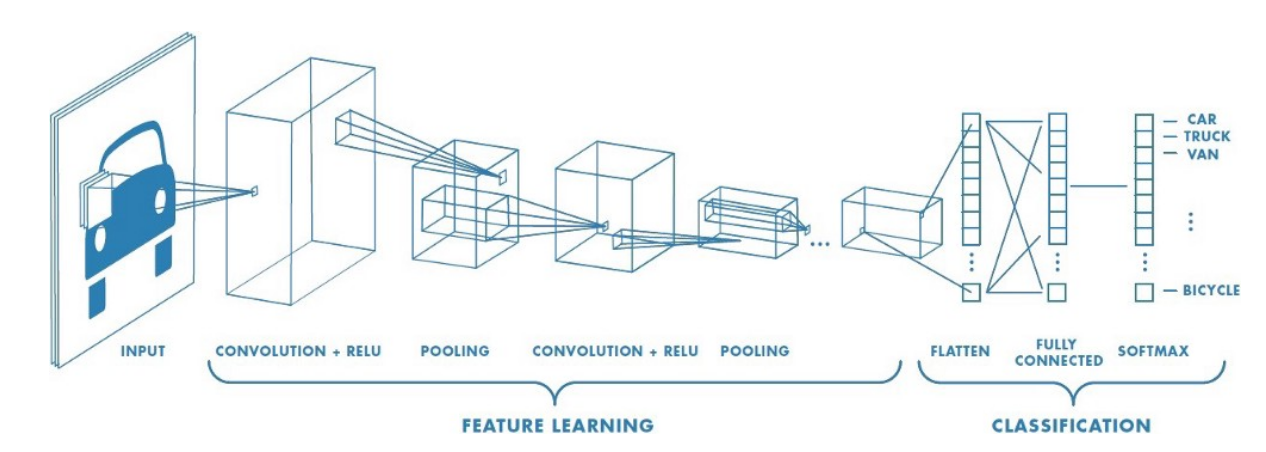Convolutional Neural Networks

Artificial intelligence has seen a tremendous growth over the last few years, The gap between machines and humans is slowly but steadily decreasing. One important difference between humans and machines is (or rather was!) with regards to human’s perception of images and sound.How do we train a machine to recognize images and sound as we do?

At this point we can ask ourselves a few questions!!!

How would the machines perceive images and sound ?

How would the machines be able to differentiate between different images for example say between a cat and a dog?

Can machines identify and differentiate between different human beings for example lets say differentiate a male from a female or identify Leonardo Di Caprio or Brad Pitt by just feeding their images to it?

Let’s attempt to find out!!!

The Colour coding system:

Lets get a basic idea of what the colour coding system for machines is

RGB decimal system: It is denoted as rgb(255, 0, 0). It consists of three channels representing RED , BLUE and GREEN respectively . RGB defines how much red, green or blue value you’d like to have displayed in a decimal value somewhere between 0, which is no representation of the color, and 255, the highest possible concentration of the color. So, in the example rgb(255, 0, 0), we’d get a very bright red. If we wanted all green, our RGB would be rgb(0, 255, 0). For a simple blue, it would be rgb(0, 0, 255).As we know all colours can be obtained as a combination of Red , Green and Blue , we can obtain the coding for any colour we want.

Gray scale: Gray scale consists of just 1 channel (0 to 255)with 0 representing black and 255 representing white. The colors in between represent the different shades of Gray.

Computers ‘see’ in a different way than we do. Their world consists of only numbers.

Every image can be represented as 2-dimensional arrays of numbers, known as pixels.

But the fact that they perceive images in a different way, doesn’t mean we can’t train them to recognize patterns, like we do. We just have to think of what an image is in a different way.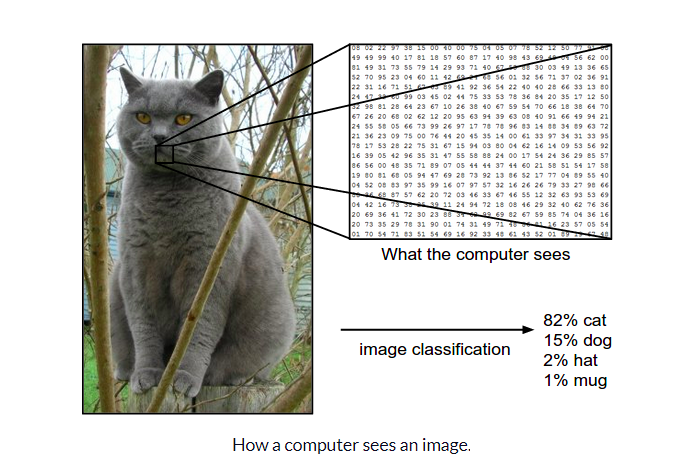Now that we have a basic idea of how images can be represented , let us try and understand The architecture of a CNN

CNN architecture

Convolutional Neural Networks have a different architecture than regular Neural Networks. Regular Neural Networks transform an input by putting it through a series of hidden layers. Every layer is made up of a set of neurons, where each layer is fully connected to all neurons in the layer before. Finally, there is a last fully-connected layer — the output layer — that represent the predictions.

Convolutional Neural Networks are a bit different. First of all, the layers are organised in 3 dimensions: width, height and depth. Further, the neurons in one layer do not connect to all the neurons in the next layer but only to a small region of it. Lastly, the final output will be reduced to a single vector of probability scores, organized along the depth dimension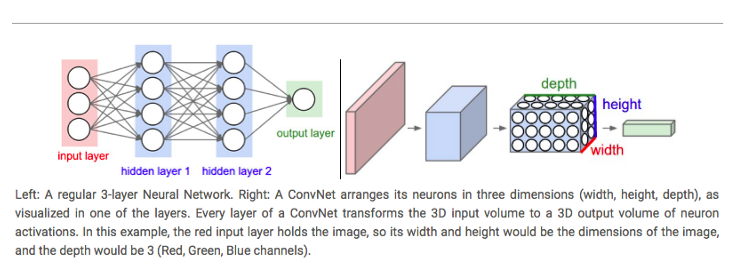A typical CNN architecture

As can be seen above CNNs have two components:

• The Hidden layers/Feature extraction part

In this part, the network will perform a series of **convolutions **and pooling operations during which the features are detected. If you had a picture of a tiger , this is the part where the network would recognize the stripes , 4 legs , 2 eyes , one nose , distinctive orange colour etc.

• The Classification part

Here, the fully connected layers will serve as a classifier on top of these extracted features. They will assign a** probability** for the object on the image being what the algorithm predicts it is.

Before we proceed any further we need to understand what is “convolution”, we will come back to the architecture later:

What do we mean by the “convolution” in Convolutional Neural Networks?

Let us decode!!!

#convolutional-neural-net #convolution #computer-vision #neural networks1624433760

## A Quick Overview of Metacat API for Discoverable Big Data

### Introduction to Metacat API

MetacatAPI is an Application Programming Interface provides an interface and helps connect the two applications and enable them to communicate with each other. Whenever we use an application or send a request, the application connects to the internet and sends the request to the server, and the server then, in response, provides the fetched data.

The data set at any organization is stored in different data warehouses like Amazon S3 (via Hive), Druid, Elasticsearch, Redshift, Snowflake, and MySql.Spark, Presto, Pig, and Hive are used to consume, process, and produce data sets. Due to numerous data sources and to make the data platform interoperate and work as a single data warehouse Metacat was built. Metacat is a metadata exploration API service. Metadata can be termed as the data about the data. Metacat explores Metadata present on Hive, RDS, Teradata, Redshift, S3, and Cassandra.

#big data engineering #blogs #a quick overview of metacat api for discoverable big data #metacat api #discoverable big data #overview